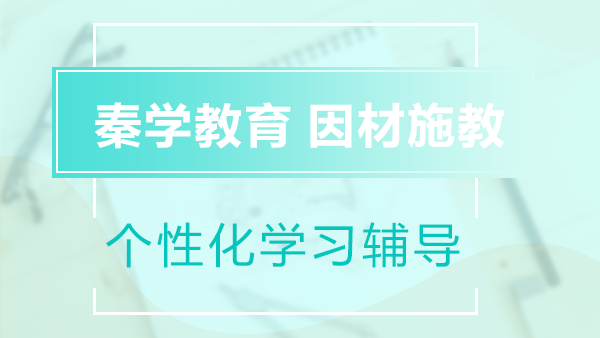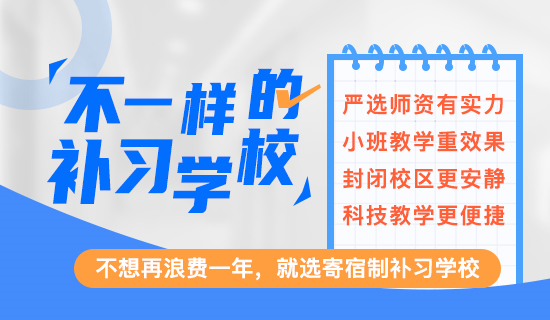2017小学四年级上册同步练习(7)，坚持就是胜利!小新2017.08.16

小学四年级的数学，开始讲究技巧，因此好多孩子都被家长逼着去上思维数学课。殊不知，这样不仅导致孩子没有时间复习基础，也让孩子没有心理负担加重。秦学教育小编建议对于基础不是很好的孩子，只要多做练习，多复习巩固，一样是可以学好数学的。一起来做做较新人教版小学四年级上册数学同步练习题吧~练习六

基础达标：

一、 口算

7×120=　　　　　　250×3=　　　　　　　　　120×5=　　　　　　　4×18=

23×4=　　　　　　 4×130= 240×3= 6×130=

140×6=　　　 　　　3×27=　　　　　　　　　 39×2=　　　　　　 380×2=

二、 计算三、 列竖式计算，并用计算器验算

343×23=　　　　　　　　　　　12×527=　　　　　　　　　　　329×23=

419×18=　　　　　　　　　　　511×15=　　　　　　　　　　　　　17×327=

拓展创新：

四、 下面的计算对吗?把不对的改正过来。五、解决问题

⒈　水果店运来125箱红富土苹果，运来桔子的箱数是苹果的11倍。运来桔子多少箱?

⒉　某防疫站上半年平均每月用电414千瓦时，下半年平均每月用电436千瓦时，这个防疫站一年共用电多少千瓦时?

⒊

216元　　　　256元　　　　　286元18066521615加入家长交流圈，添加微信号18066521615+年级学科资料